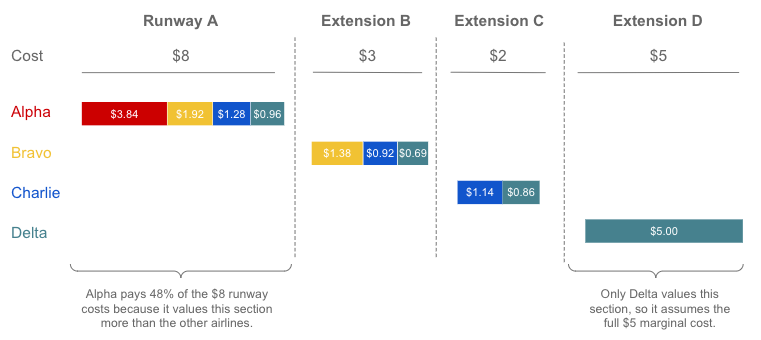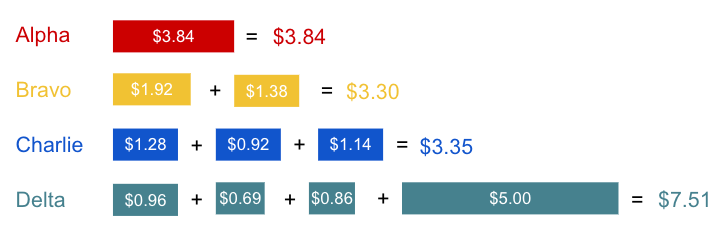# The Airport Problem Revisted

## Relative Need Splitting Solution

• Alpha =100% of runway needs = 1/1 = 12/12
• Bravo = 50% of runway needs = 1/2 = 6/12
• Charlie = 33% of runway needs = 1/3 = 4/12
• Delta = 25% of runway needs = 1/4 = 3/12Cost split when considering the relative value of a section of runway to each’s overall needs.Total cost per airline considering the relative value of a section of runway to each’s overall needs.

## Relative Costs Splitting Solution

• Alpha =100% of runway costs = \$8/\$8 = 10,296 / 10,296
• Bravo = 72.7% of runway needs = \$8/\$11 = 7,488 / 10,296
• Charlie = 61.5% of runway needs = \$8/\$13 = 6,336 / 10,296
• Delta = 44.4% of runway needs = \$8/\$18 = 4,576 / 10,296

--

--